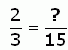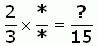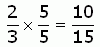SEARCH HOMEMath Central Quandaries & QueriesQuestion from marlene, a parent: find equivalent fractions with a denominator of 8 1/2Hi Marlene. Let me know if I am wrong, but I think your question is asking for a fraction equivalent to 1/2 with a denominator of 8.

You can find an equivalent fraction to any fraction by multiplying the top (numerator) and bottom (denominator) by any value as long as it is the same value.

I will show you an example that you can follow to solve your own question.

Example: Find an equivalent fraction for 2/3 whose denominator is 15.

So the question is asking this:What is the question mark?

From what I told you, you can find an equivalent fraction by multiplying the top (2) by some value and multiplying the bottom (3) by some value. They have to be the same value.

So if we write an asterisk (*) in place of this mystery value, we can say this:So for the denominators, I know that 3 x 5 = 15, so the * must be a 5. I can write that in both places and finish the problem: